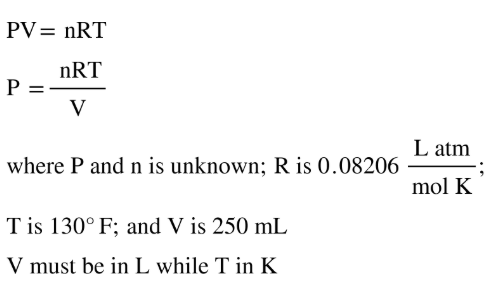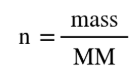# Problem: An aerosol spray can with a volume of 250 mL contains 2.30 g of propane gas (C3H8) as a propellant.The can says that exposure to temperatures above 130 oF may cause the can to burst. What is the pressure in the can at this temperature?

###### FREE Expert Solution
• Use the ideal gas equation to find the pressure where we have to convert the provided mass into moles
• Ideal gas equation will appear as:• Calculating for moles (n):MM of C3H8:

C - 12.01 (3) = 36.03

H - 1.01 (8) = 8.08

36.03 + 8.08 = 44.11 g/mol

93% (338 ratings)###### Problem Details

An aerosol spray can with a volume of 250 mL contains 2.30 g of propane gas (C3H8) as a propellant.

The can says that exposure to temperatures above 130 oF may cause the can to burst. What is the pressure in the can at this temperature?